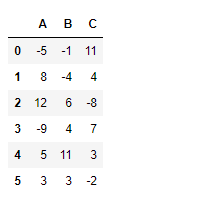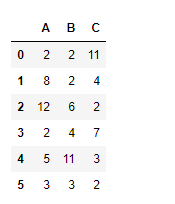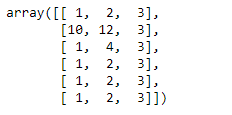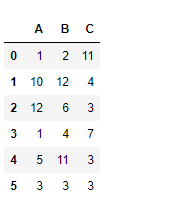GFG App
Open AppBrowser
Continue

# Python | Pandas dataframe.clip_lower()

Python is a great language for doing data analysis, primarily because of the fantastic ecosystem of data-centric python packages. Pandas is one of those packages and makes importing and analyzing data much easier.

Pandas` dataframe.clip_lower()` is used to trim values at specified input threshold. We use this function to trim all the values below the threshold of the input value.

Syntax: DataFrame.clip_lower(threshold, axis=None, inplace=False)

Parameters:
threshold : numeric or array-like
`float `: every value is compared to threshold.
`array-like` : The shape of threshold should match the object it’s compared to. When self is a Series, threshold should be the length. When self is a DataFrame, threshold should 2-D and the same shape as self for axis=None, or 1-D and the same length as the axis being compared.
axis : Align self with threshold along the given axis.
inplace : Whether to perform the operation in place on the data.

Returns: clipped : same type as input

Example #1: Use `clip_lower()` function to trim values of a data frame below a given threshold value.

 `# importing pandas as pd ` `import` `pandas as pd ` ` `  `# Creating a dataframe using dictionary ` `df ``=` `pd.DataFrame({``"A"``:[``-``5``, ``8``, ``12``, ``-``9``, ``5``, ``3``], ` `                   ``"B"``:[``-``1``, ``-``4``, ``6``, ``4``, ``11``, ``3``],  ` `                   ``"C"``:[``11``, ``4``, ``-``8``, ``7``, ``3``, ``-``2``]}) ` ` `  `# Printing the data frame for visualization ` `df `Now trim all the values below 2 to 2.

 `# Clip all values below 2 ` `df.clip_lower(``2``) `

Output :Example #2: Use `clip_lower()` function to clips values in a dataframe with specific value for each cell of the dataframe.

For this purpose, we can use a numpy array, but the shape of array must be same as that of the dataframe.

 `# importing pandas as pd ` `import` `pandas as pd ` ` `  `# Creating a dataframe using dictionary ` ` `  `df ``=` `pd.DataFrame({``"A"``:[``-``5``, ``8``, ``12``, ``-``9``, ``5``, ``3``],  ` `                   ``"B"``:[``-``1``, ``-``4``, ``6``, ``4``, ``11``, ``3``], ` `                   ``"C"``:[``11``, ``4``, ``-``8``, ``7``, ``3``, ``-``2``]}) ` ` `  `# lower limit for each individual column element. ` `limit ``=` `np.array([[``1``, ``2``, ``3``], [``10``, ``12``, ``3``], [``1``, ``4``, ``3``], ` `                  ``[``1``, ``2``, ``3``], [``1``, ``2``, ``3``], [``1``, ``2``, ``3``]]) ` ` `  `# Print lower_limit ` `limit `Now apply these limits on the dataframe

 `# applying different limit value  ` `# for each cell in the dataframe ` `df.clip_lower(limit) `

Output :Each cell value has been trimmed based on the corresponding lower limit applied.

My Personal Notes arrow_drop_up